﻿ 低速机电控喷油器电磁阀换热特性计算
 舰船科学技术2022, Vol. 44Issue (10): 89-92    DOI: 10.3404/j.issn.1672-7649.2022.10.017PDF

, 董垚2, 刘承建3, 陈康3, 郑津4, 涂天华1,3
1. 船舶与海洋工程动力系统国家工程实验室，重庆 402160;
2. 重庆大学 机械传动国家重点实验室，重庆 400044;
3. 重庆红江机械有限责任公司，重庆 402160;
4. 重庆大学 弘深学院机械实验班，重庆 400044

Simulation of heat transfer characteristics of solenoid valve in the low-speed diesel engine's electronic injector
, DONG Yao2, LIU Chengjian3, CHEN Kang3, ZHENG Jin4, TU Tian-hua1,3
1. National Engineering Laboratory for Marine and Ocean Engineering Power System, Chongqing 402160, China;
2. State Key Laboratory of Mechanical Transmission, Chongqing University, 400044 Chongqing, China;
3. Chongqing Hongjiang Machinery Co. Ltd., Chongqing 402160, China;
4. Hongshen Honors School, Chongqing University, Chongqing 400044, China
Abstract: To study the hydraulics and heat transfer characteristics of the solenoid valve of the Low-speed diesel engine’s electronic controlled injector, one valve assembly is taken as the research object. One the basis of satisfying the mechanical properties of the solenoid valve, a 3-D model is established and Fluent is used to analyze the heat transfer of fluid-structure interaction coupling of the components. Analysis results shows when the inlet temperature of lubricating oil is 55℃,the outlet is 78.7℃, and the inlet temperature of heavy oil is 200℃, the outlet is 198.8℃. The temperature of valve near the heavy oil increases gradually, and the temperature rise of the solenoid valve is greatly affected by the heavy oil. The maximum temperature of the solenoid valve is 93.42℃,which is far less than the limit temperature.The structure meets the requirements of working performance, the solenoid valve’s heat exchange effect is reasonable. Through the analysis process of conjugate heat transfer, this paper provides guidance for the design of the solenoid valve of the low-speed diesel engine’s electronic controlled injector.
Key words: low-speed diesel engine     solenoid valve     fluid-structure interaction     conjugate heat transfer
0 引　言

1 电磁阀结构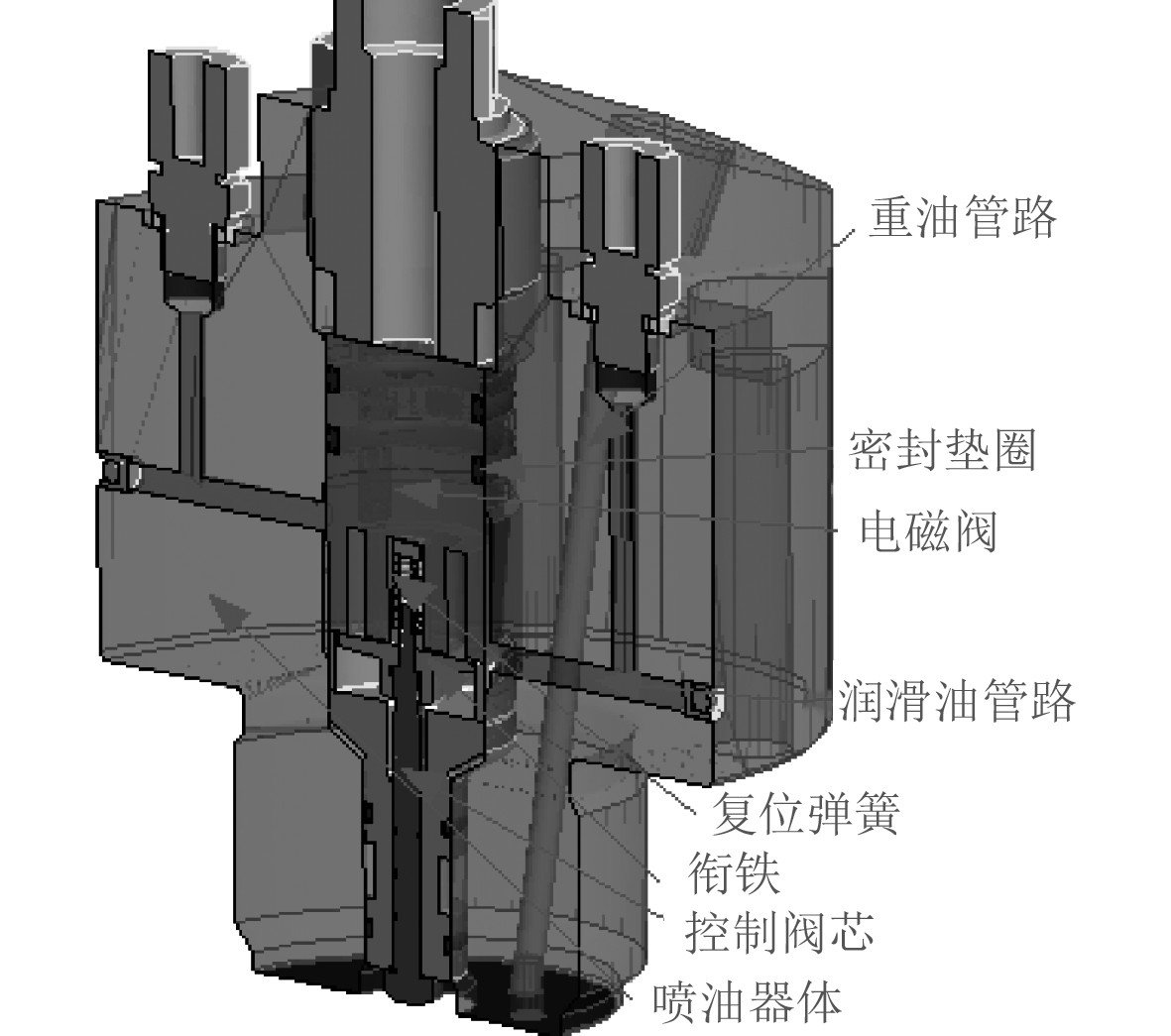图 1 电磁阀组件剖面图 Fig. 1 Component section of solenoid valve
2 电磁阀传热理论 2.1 传热理论

 $\frac{1}{r}\times \frac{\partial }{\partial r}\left(kr\frac{\partial T}{\partial r}\right)+\frac{1}{{r}^{2}}\times \frac{\partial }{\partial \phi }\left(k\frac{\partial T}{\partial \phi }\right) +\frac{\partial }{\partial z}\left(k\frac{\partial T}{\partial z}\right)+\varnothing =0。$ (2.1)

 ${q}={h}({T}_{\infty }-{T}_{s})。$ (2.2)

 ${E}=\mathrm{\epsilon }\mathrm{\sigma }{T}_{s}^{4}。$ (2.3)

 $\frac{\partial }{\partial t}\rho {u}_{i}+\frac{\partial }{\partial {x}_{i}}\rho {u}_{i}{u}_{j}=-\frac{\partial p}{\partial {x}_{i}}+\frac{\partial }{\partial {x}_{i}}\mu \left(\frac{\partial {u}_{i}}{\partial {x}_{i}}+\frac{\partial {u}_{i}}{\partial {x}_{j}}\right)+{F}_{i}，$ (2.4)
 $\frac{\partial }{\partial t}\mathrm{\rho }{E}+\frac{\partial }{\partial {x}_{i}}{u}_{i}\left(\rho E+p\right)=\frac{\partial }{\partial {x}_{i}}k\frac{\partial T}{\partial {x}_{i}}。$ (2.5)

3 共轭耦合计算 3.1 仿真模型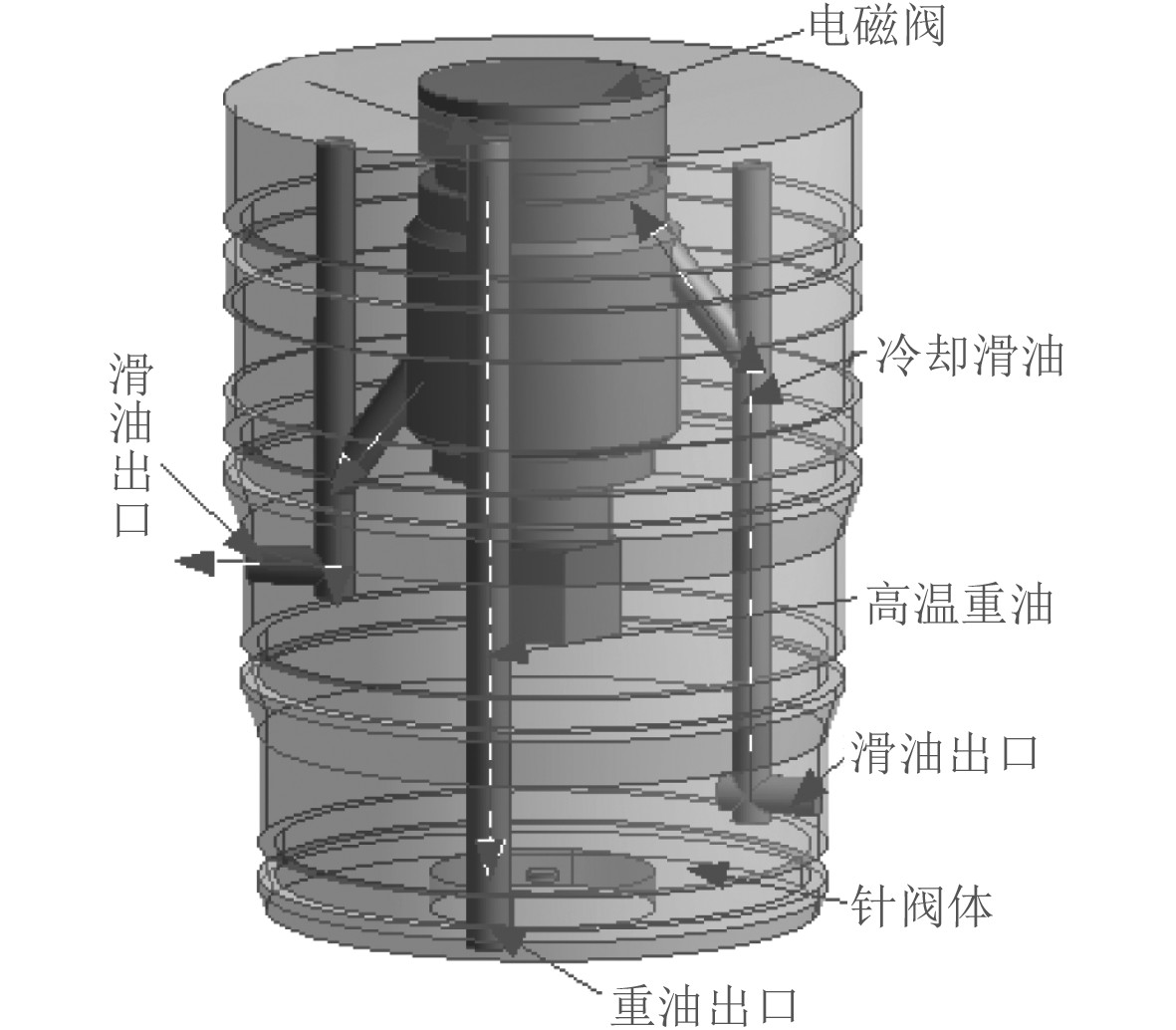图 2 计算模型 Fig. 2 Computational model
3.2 计算网格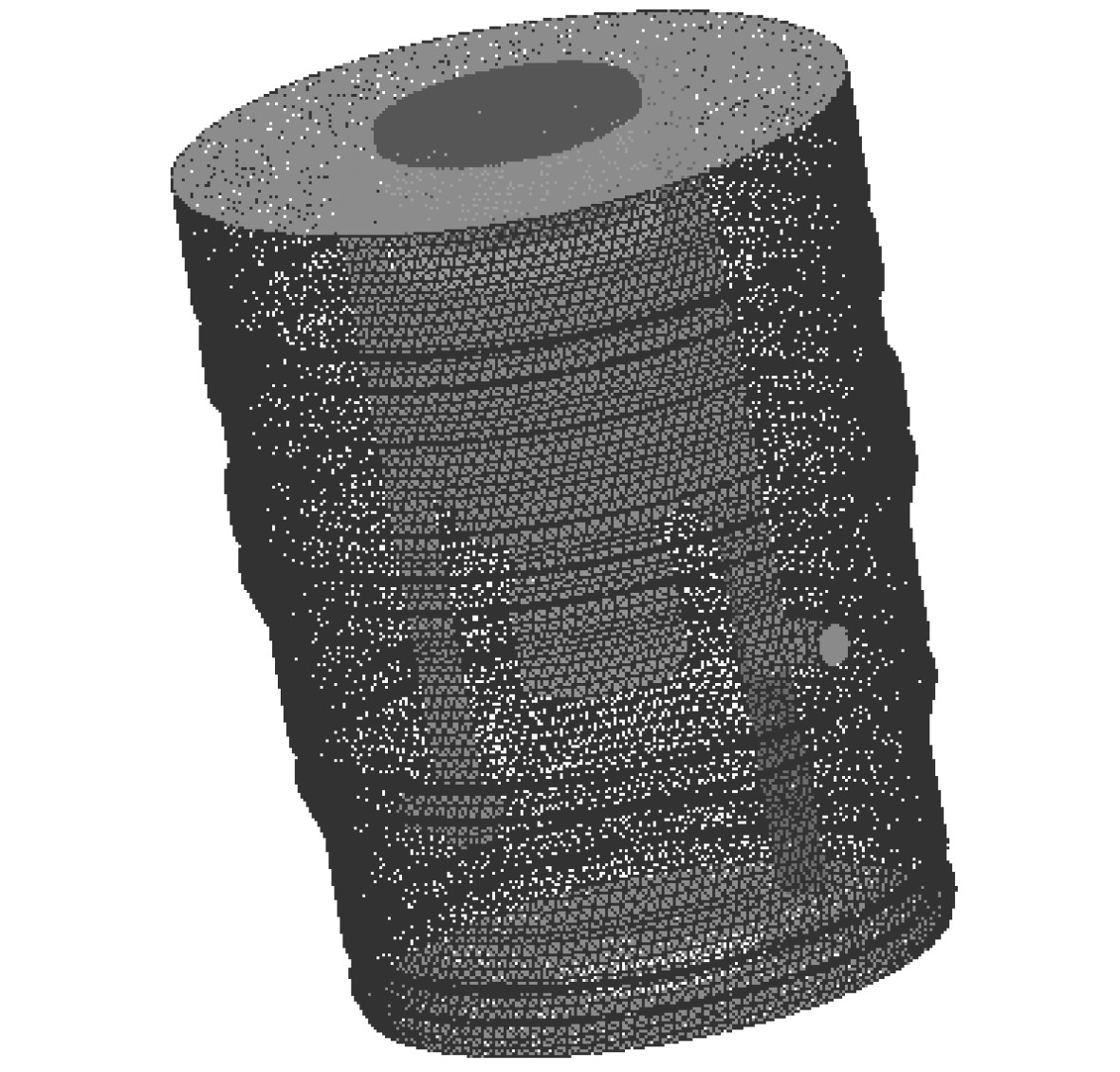图 3 模型网格 Fig. 3 Model mesh
3.3 边界条件及物质属性表 1 材料属性及边界 Tab.1 Material properties and boundaries
3.4 湍流模型

Fluent提供了丰富的湍流模型，如标准k-ε模型、RNG k-ε模型、低雷诺数Wilcox模型、低雷诺数Menter、代数雷诺应力模型、微分雷诺应力模型、微分雷诺通量模型及LES模型、DES模型等[6-7]。针对燃油系统电磁阀冷却仿真的问题，采用SST k-ω模型进行计算分析，该湍流模型包含修正的湍流粘性公式，并且考虑了湍流剪切应力产生的效应，考虑了湍流剪切应力的SST k-ω模型不会对涡流粘度造成过度预测，对设置近壁面参数计算敏感，计算结果准确度高。

4 共轭换热分析表 2 流域计算结果 Tab.2 Fluid calculation results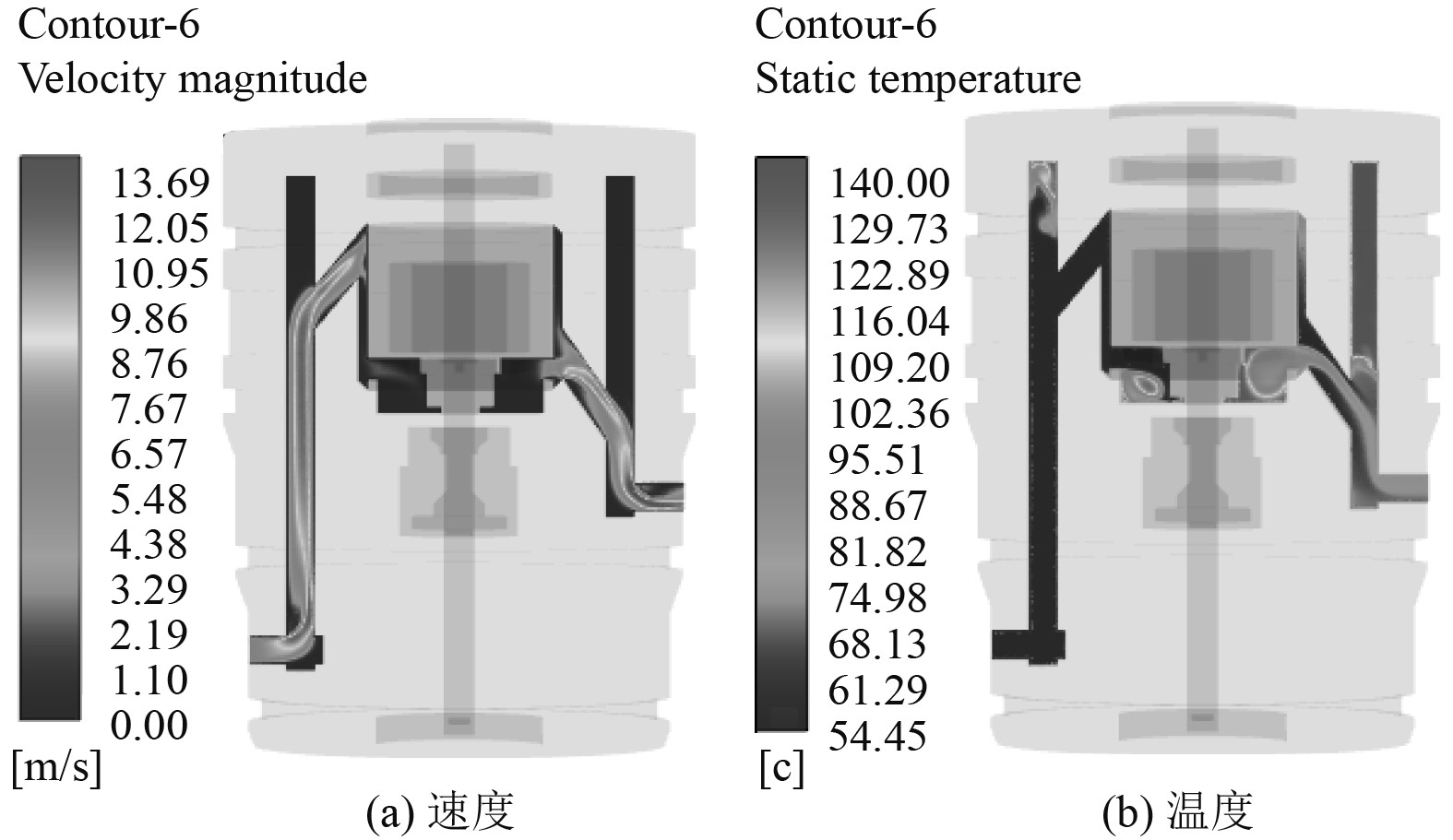图 4 滑油计算云图 Fig. 4 Calculation results of lubricating oil表 3 共轭换热温度结果 Tab.3 Conjugate heat transfer temperature results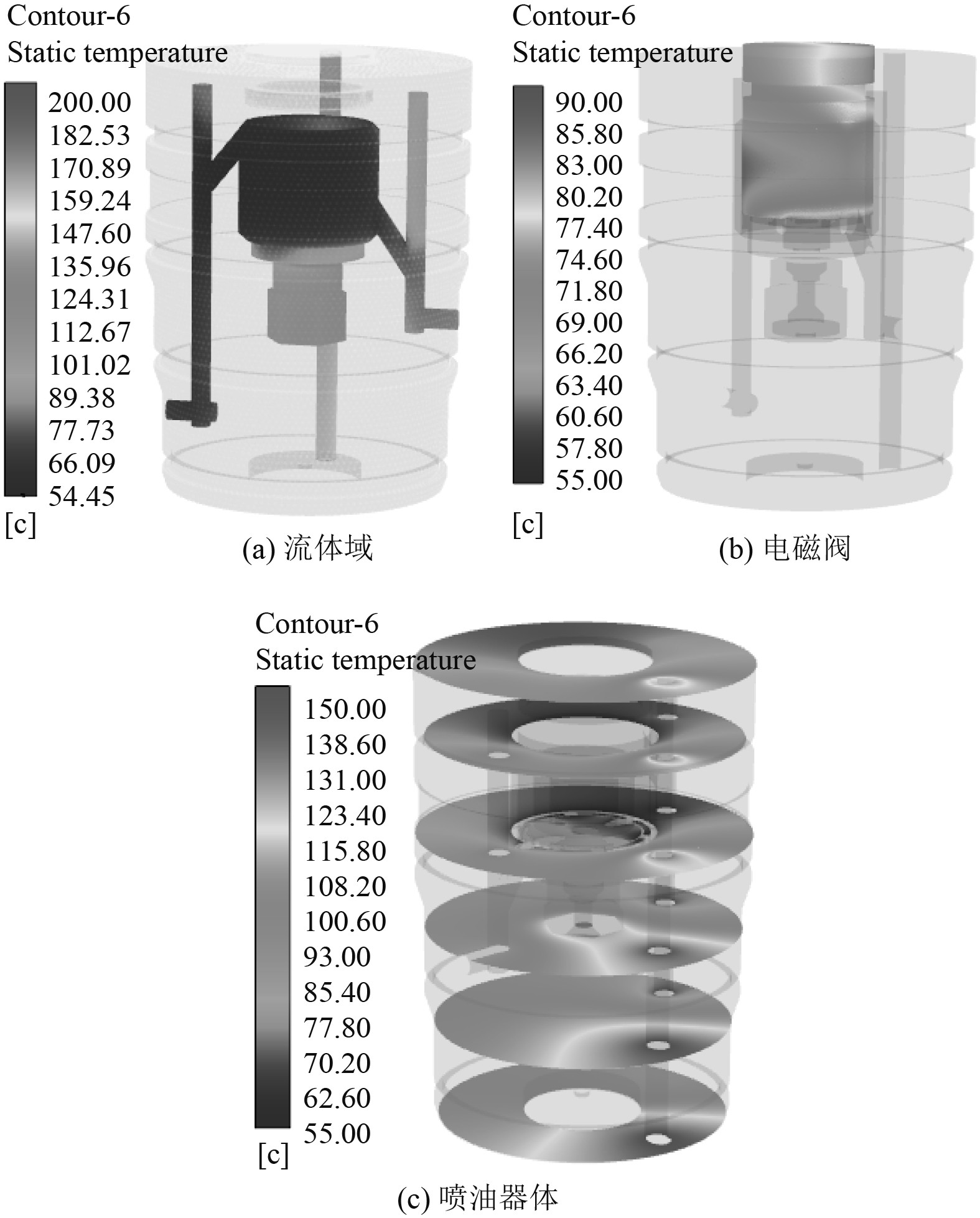图 5 共轭换热计算云图 Fig. 5 Cloud of conjugate heat transfer calculation
5 结　语

1） 滑油流动方向温度不断升高，重油在流动方向温度下降，电磁阀靠近重油附近温度逐渐升高。

2） 电磁阀的温升来源线圈和重油散热，受重油的影响较大。

3） 电磁阀最高温度93.42℃，远小于极限温度，换热效果较好，其结构满足工作性能要求。

4） 流固热共轭耦合换热分析，为船用低速机喷油器电磁阀设计提供指导。

  常浩. 一种汽车机油泵电磁阀的稳态温度场分析[D]. 上海: 上海交通大学, 2015  刘鹏. 电控燃油喷射系统复合磁路高速电磁阀设计与特性研究[D]. 哈尔滨: 哈尔滨工程大学, 2018  杨世铭, 陶文铨. 传热学[M]. 北京: 北京高等教育出版社, 2006  张师帅. 计算流体动力学及其应用[M]. 武汉: 华中科技大学出版社, 2010: 5-8.  陈继涛, 宋海燕. 基于Fluent的柴油机冷却环道流动特性分析[J]. 液压与气动, 2019(10): 44-49. DOI:10.11832/j.issn.1000-4858.2019.10.008  王福军. 计算流体动力学分析CFD软件原理与应用[M]. 北京: 清华大学出版社, 2004.  MENTER F R. Two-equation eddy-viscosity turbulence models for engineering applications[J]. Aiaa Journal, 1994, 32(8): 1598-1605.  刘友宏, 丁玉林, 常正则, 等. 发动机矢量喷管作动器电磁阀非稳态热分析[J]. 科学技术与工程, 2016(16): 285-289. DOI:10.3969/j.issn.1671-1815.2016.16.049